﻿ 基于GNSS的地基增强系统信号质量监测阈值设计文章快速检索 高级检索
 大地测量与地球动力学2021, Vol. 41Issue (7): 690-694  DOI: 10.14075/j.jgg.2021.07.006### 引用本文SUN Hao, SHI Xiaozhu, LIU Haiying, et al. Design of SQM Threshold for Ground-Based Augmentation System Based on GNSS[J]. Journal of Geodesy and Geodynamics, 2021, 41(7): 690-694.### Foundation support

Open Fund of State Key Laboratory of Air Traffic Management System and Technology, No.SKLATM201903; Aviation Science Foundation, No.201908052002.

### 第一作者简介

SUN Hao, postgraduate, majors in integrity of GNSS/GBAS, E-mail: wsduke@foxmail.com.

### 文章历史

1. 南京航空航天大学航天学院，南京市将军大道29号，211106;
2. 空中交通管理系统与技术国家重点实验室，南京市永智路8号，210016

1 基于高斯分布的SQM阈值模型

SQM算法是GBAS系统完好性算法中的重要组成部分，目的是及时发现测距信号中存在的异常情况。接收信号功率监测和码/载波分歧监测作为SQM算法的2个重要功能，其阈值设定是否准确至关重要

1.1 载噪比阈值模型

GBAS地面站对接收机接收到的载噪比进行平均处理，对于每个信道，载噪比平均值为：

 $\begin{array}{l} {\rm{CN}}{{\rm{R}}_{{\rm{avg}}, (i, j)}} = \frac{1}{2}\left( {{\rm{CN}}{{\rm{R}}_{(i, j)}}(k) + } \right.\\ \;\;\;\;\;\;\;\;\;\;\;\left. {{\rm{CN}}{{\rm{R}}_{(i, j)}}(k - 1)} \right) \end{array}$ (1)

 ${\rm{CN}}{{\rm{R}}_{{\rm{avg}}, (i, j)}} \sim N\left( {{\mu _{{\rm{cnr}}, (i, j)}}, {\sigma _{{\rm{cnr}}, (i, j)}}} \right)$ (2)

 ${T_{{\rm{cnr}}, (i, j)}} = {\mu _{(i, j)}} - K{\sigma _{(i, j)}}$ (3)

1.2 CCD阈值模型

 $\begin{array}{l} {\rm{CC}}{{\rm{D}}_{m, n}}(k) = \frac{{{\tau _d} - {T_s}}}{{{\tau _d}}}{\rm{CC}}{{\rm{D}}_{m, n}}(k - 1) + \\ \;\;\;\;\;\;\;\;\;\;\;\;\;\;\;\;\frac{1}{{{\tau _d}}}{\rm{d}}{Z_{m, n}}(k) \end{array}$ (4)
 ${Z_{m, n}}(k) = {\rho _{m, n}}(k) - {\varphi _{m, n}}(k)$ (5)

 ${\rm{CC}}{{\rm{D}}_{(i, j)}} \sim N\left( {{\mu _{{\rm{ccd}}, (i, j)}}, {\sigma _{{\rm{ccd}}, (i, j)}}} \right)$ (6)

 ${T_{{\mathop{\rm ccd}\nolimits} , (i, j)}} = {\mu _{(i, j)}} \pm 6{\sigma _{(i, j)}}$ (7)

2 基于稳定分布的SQM阈值模型 2.1 稳定分布与最大似然估计

 $\mathit{\Phi } (t) = \exp \left( {{\rm{j}}\delta t - |\gamma t{|^a}\left( {1 - {\rm{j}}\beta {\mathop{\rm sgn}} (t)\tan \left( {\frac{{\pi \alpha }}{2}} \right)} \right)} \right)$ (8)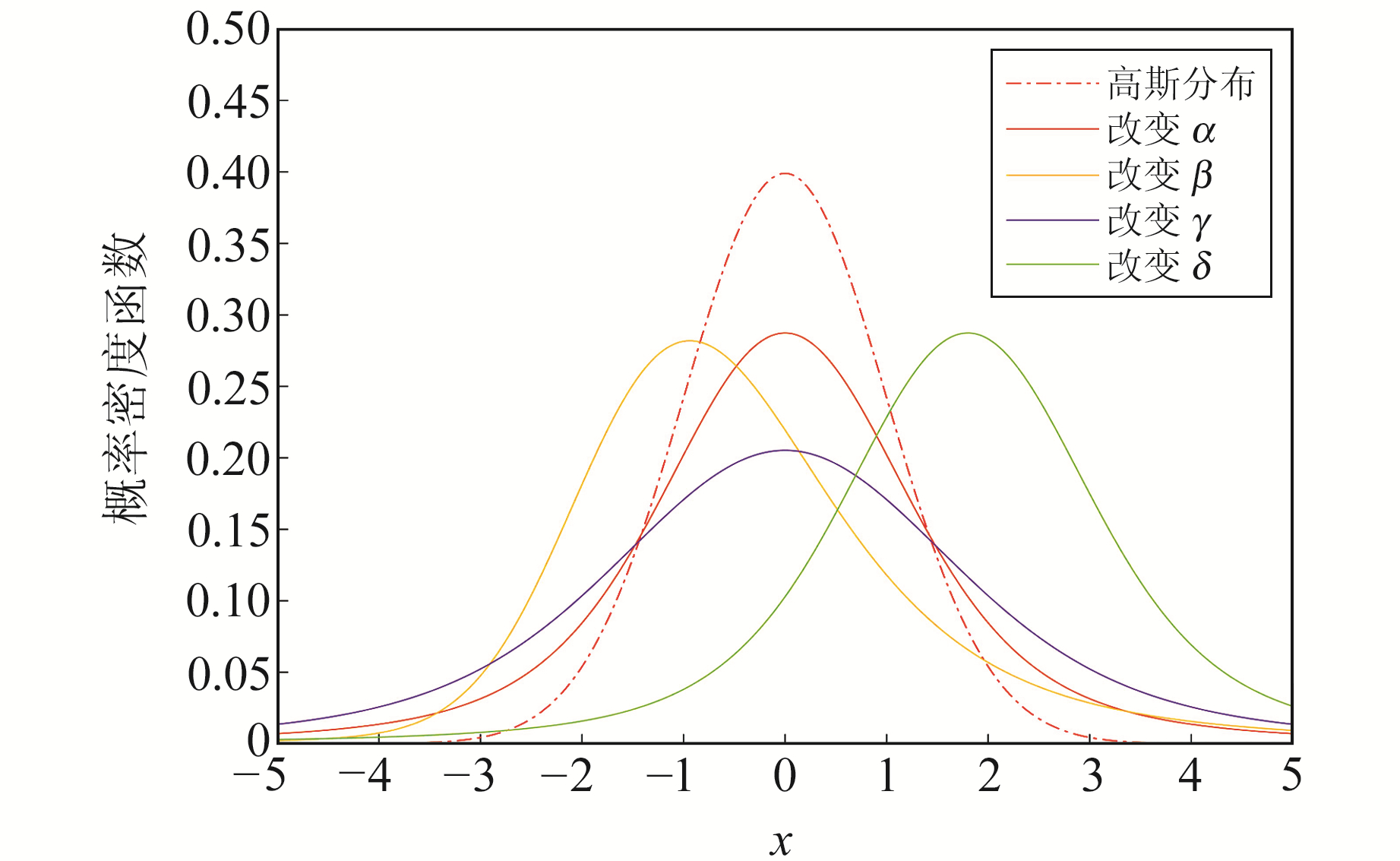图 1 稳定分布参数对概率密度函数的影响 Fig. 1 The influence of stable distribution parameters on probability density function

 $L(\theta ) = \sum\limits_{i = 1}^n {\log } f\left( {{x_i};\theta } \right)$ (9)

 $\frac{{\partial L(P)}}{{\partial \alpha }} = \frac{{\partial L(P)}}{{\partial \gamma }} = \frac{{\partial L(P)}}{{\partial \delta }} = 0$ (10)
2.2 基于稳定分布的误差模型

 ${\rm{CN}}{{\rm{R}}_{(i, j)}} \sim S\left( {{\alpha _{(i, j)}}, {\beta _{(i, j)}}, {\gamma _{(i, j)}}, {\delta _{(i, j)}}} \right)$ (11)

 ${T_{{\rm{cnr}}, \max }} = {Q_s}\left( {{P_{{\rm{cnr}}}};{\alpha _{{\rm{cnr}}}};{\beta _{{\rm{cnr}}}};{\sigma _{{\rm{cnr}}}};{\delta _{{\rm{cnr}}}}} \right)$ (12)

 ${T_{{\mathop{\rm ccd}\nolimits} , \max }} = {Q_s}\left( {P;{\alpha _{{\rm{ccd}}}};{\beta _{{\rm{ccd}}}};{\sigma _{{\rm{ccd}}}};{\delta _{{\rm{ccd}}}}} \right)$ (13)
 ${T_{{\rm{ccd}}, \min }} = Qs\left( {1 - P;{\alpha _{{\rm{ccd}}}};{\beta _{{\rm{ced}}}};{\sigma _{{\rm{ccd}}}};{\delta _{{\rm{ccd}}}}} \right)$ (14)

3 实验验证及分析 3.1 实验过程及数据拟合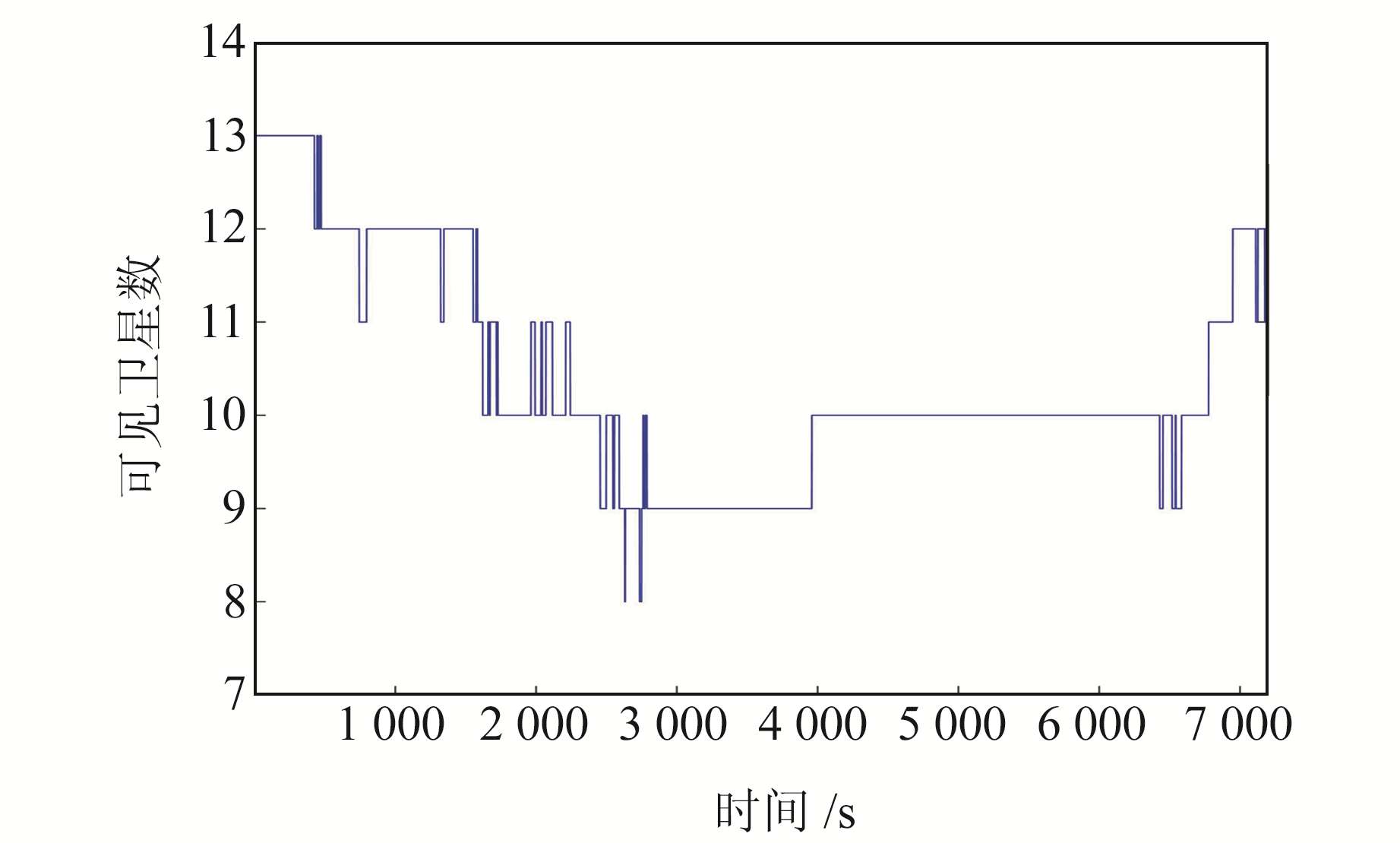图 2 可见卫星数 Fig. 2 Number of visible satellites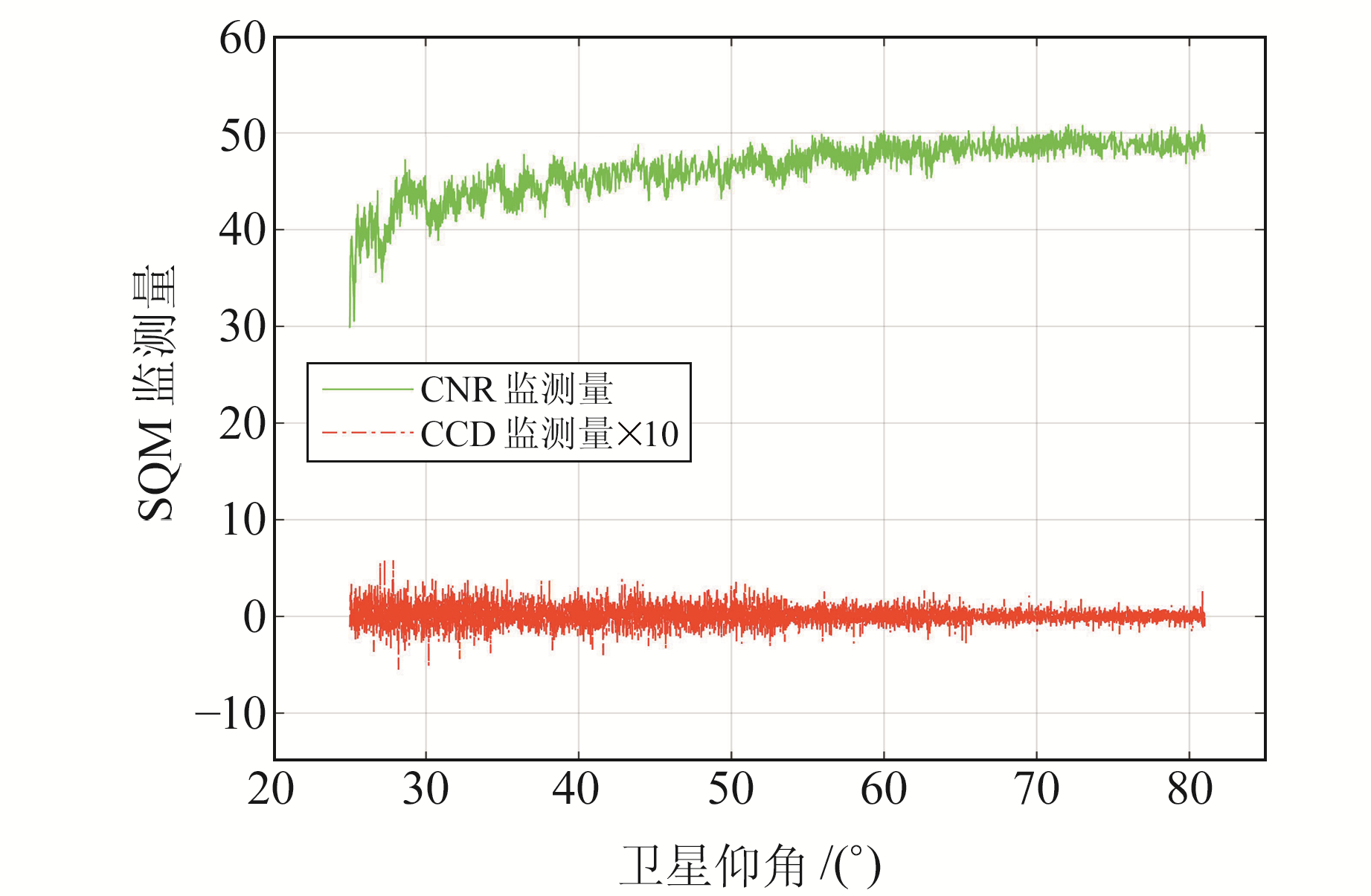图 3 SQM监测量与对应卫星仰角 Fig. 3 SQM monitoring quantities and corresponding satellite elevation angle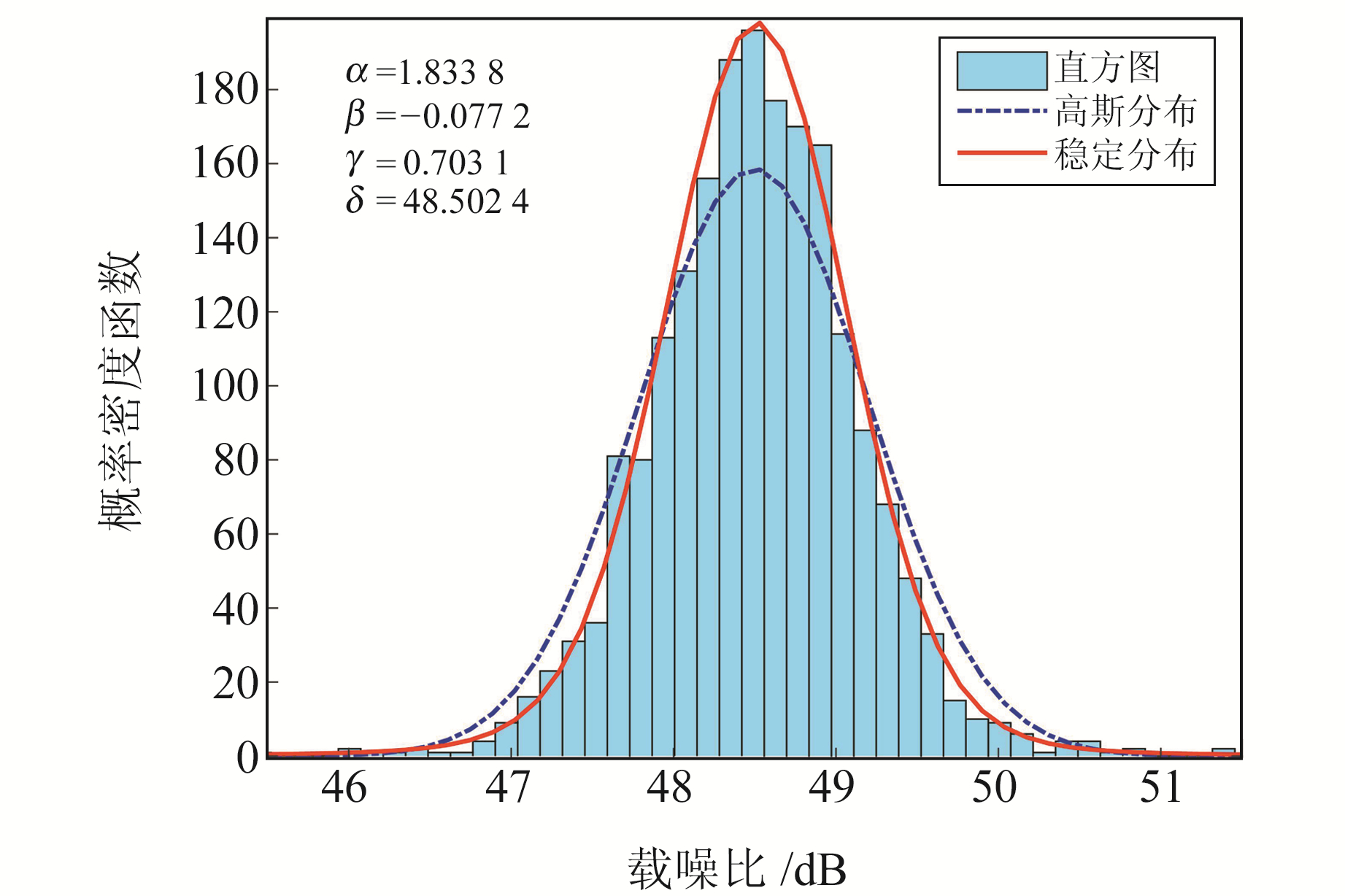图 4 载噪比数据拟合 Fig. 4 CNR data fitting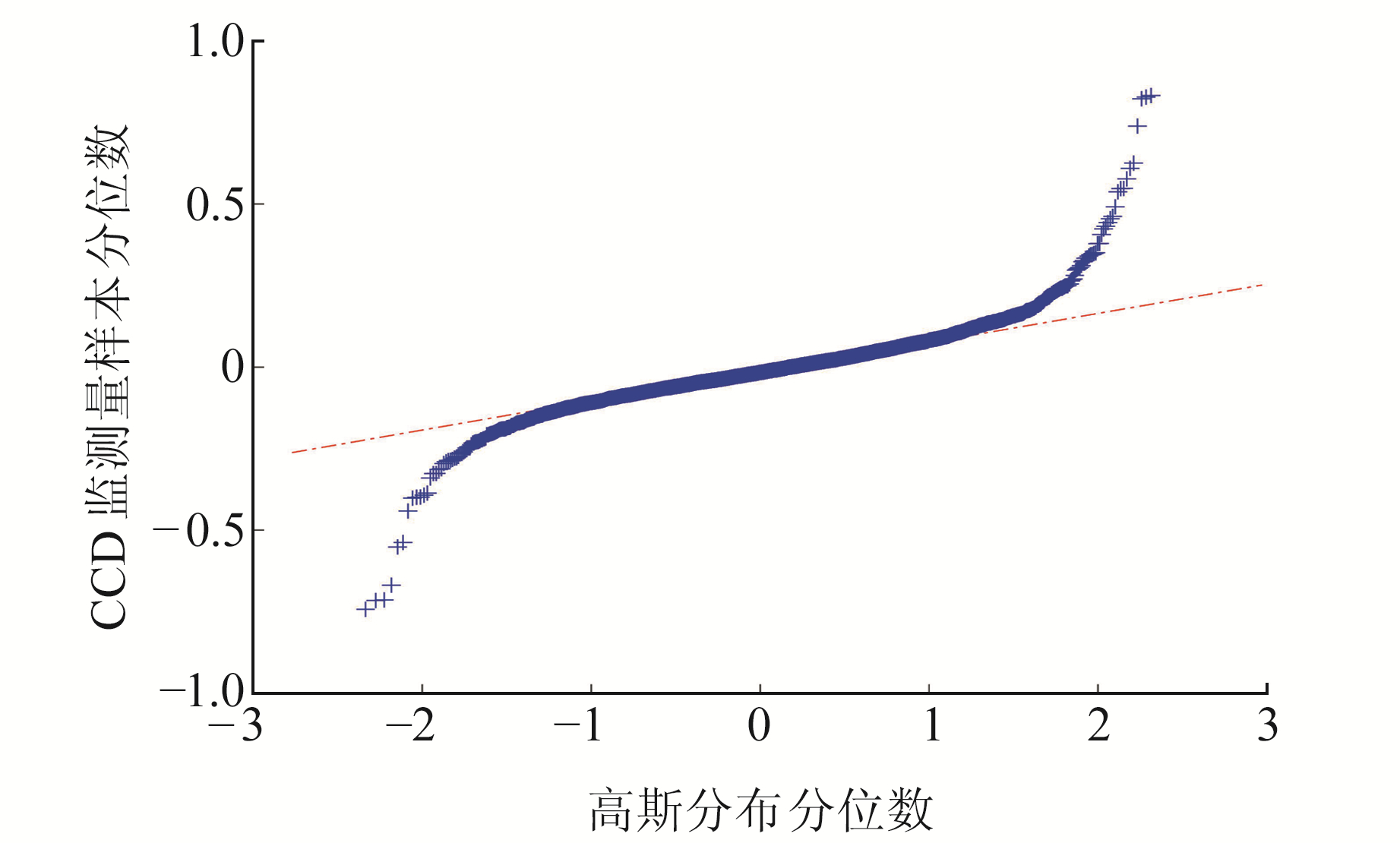图 5 高斯分布下CCD样本Q-Q图 Fig. 5 Q-Q plot of CCD sample under Gaussian distribution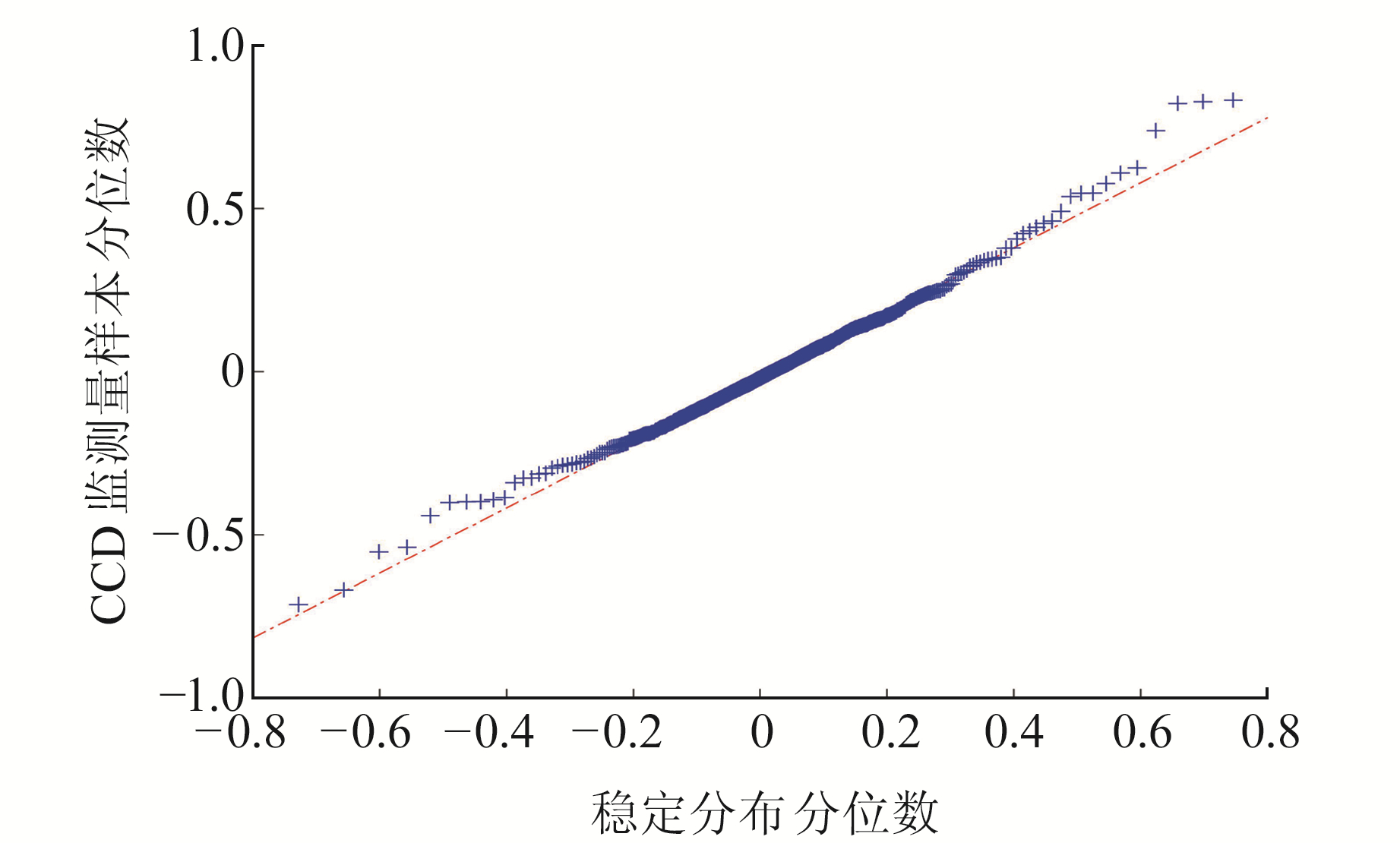图 6 稳定分布下CCD样本Q-Q图 Fig. 6 Q-Q plot of CCD sample under stable distribution表 1 CCD参数估计结果 Tab. 1 Estimation result of CCD parameters
3.2 实验结果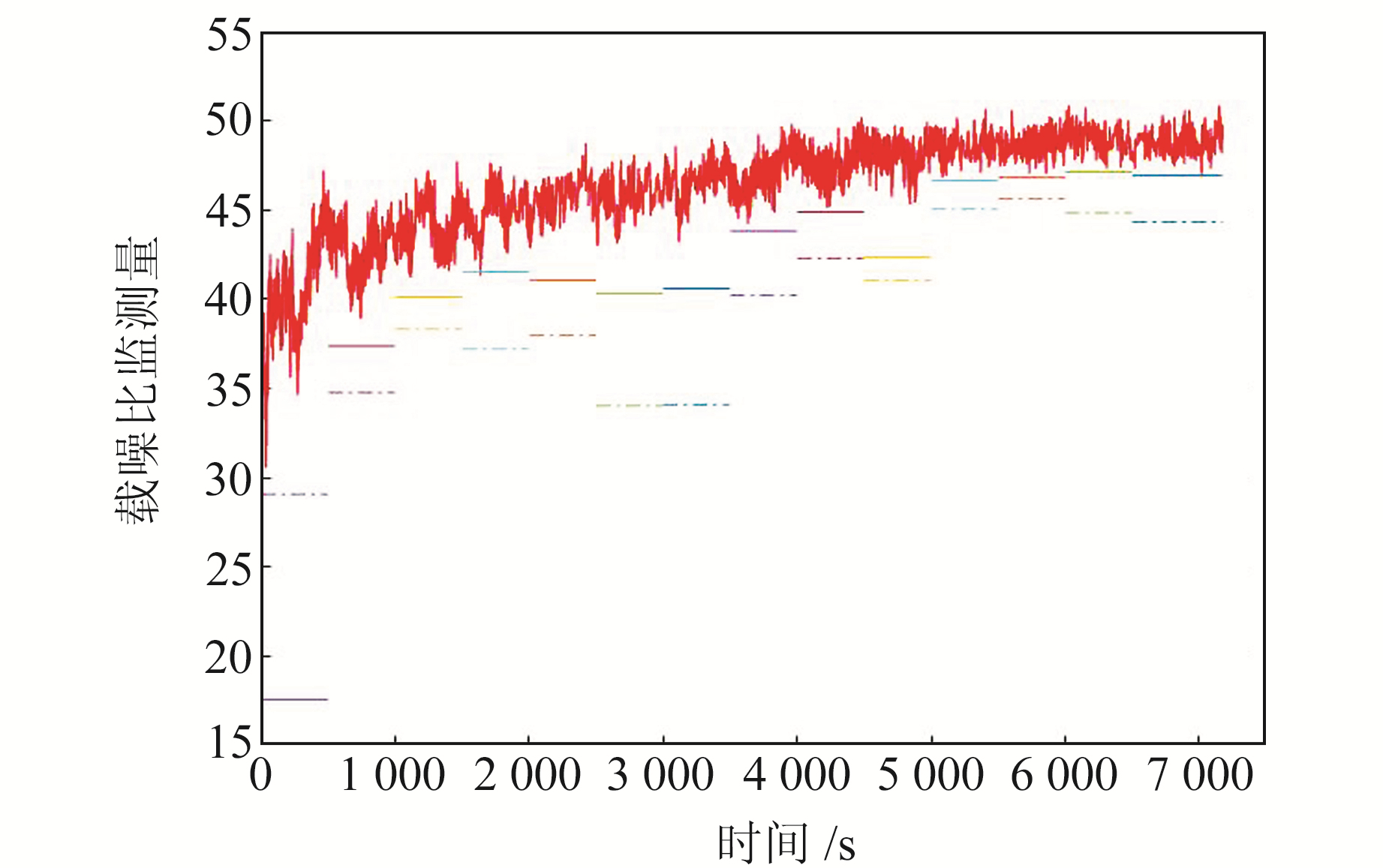图 7 观测时段内载噪比及阈值 Fig. 7 CNR and threshold during observation time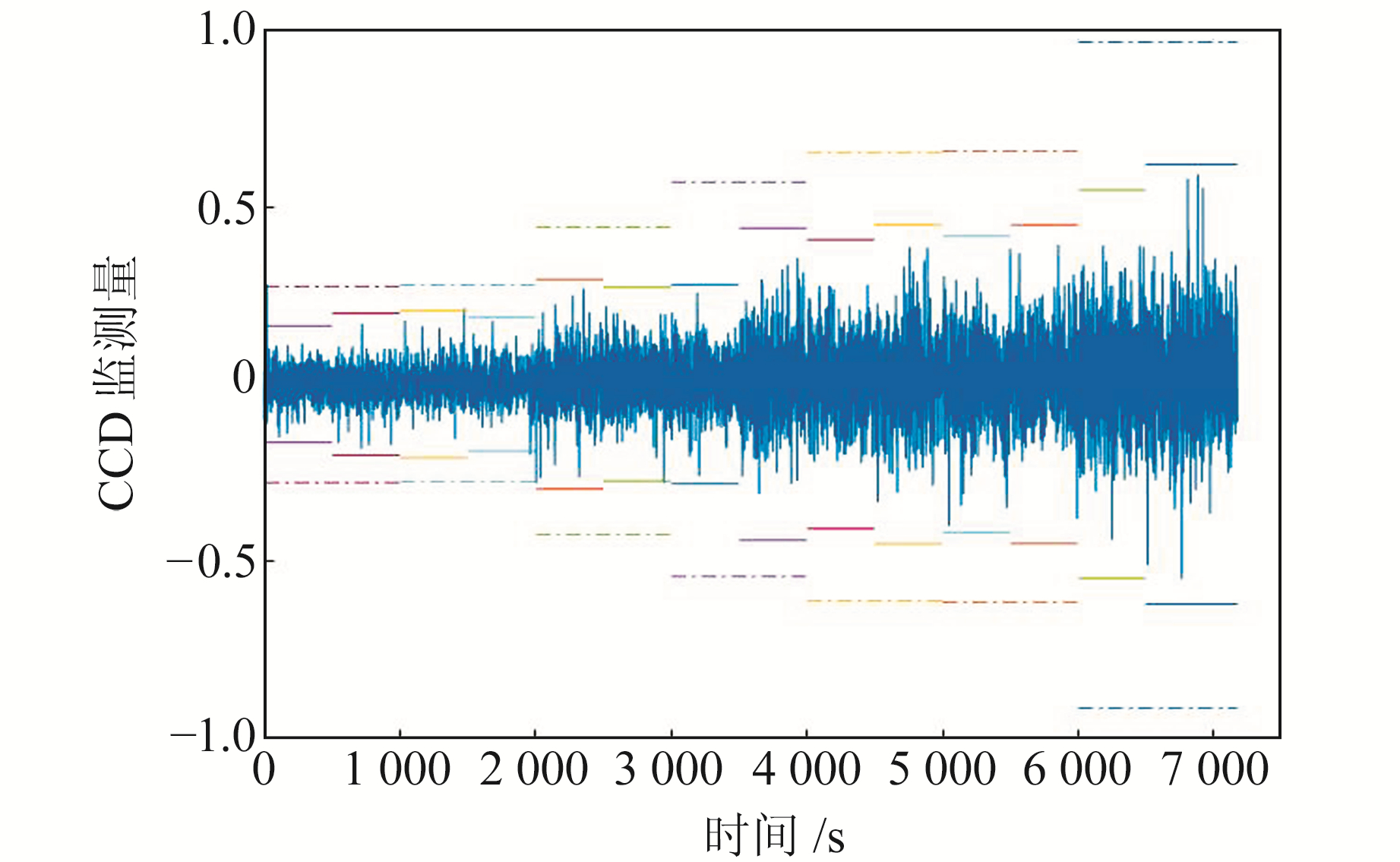图 8 观测时段内CCD监测量及阈值 Fig. 8 CCD monitoring quantities and threshold during observation time表 2 两种分布计算的CCD/CNR阈值均值 Tab. 2 The average CCD/CNR threshold calculated by 2 methods
4 结语

  刘海颖, 王惠南, 陈志明. 卫星导航原理与应用[M]. 北京: 国防工业出版社, 2013 (Liu Haiying, Wang Huinan, Chen Zhiming. Principle and Application of Satellite Navigation[M]. Beijing: National Defense Industry Press, 2013) (0)  López-Lago M, Serna J, Casado R, et al. Present and Future of Air Navigation: PBN Operations and Supporting Technologies[J]. International Journal of Aeronautical and Space Sciences, 2020, 21: 451-468 DOI:10.1007/s42405-019-00216-y (0)  Nikiforov I. From Pseudorange Overbounding to Integrity Risk Overbounding[J]. Navigation, 2019, 66(2): 417-439 DOI:10.1002/navi.303 (0)  Jiang Y P, Milner C, Macabiau C. Code Carrier Divergence Monitoring for Dual-Frequency GBAS[J]. GPS Solutions, 2017, 21(2): 769-781 DOI:10.1007/s10291-016-0567-4 (0)  Teunissen P J G, Montenbruck O. Springer Handbook of Global Navigation Satellite Systems[Z]. Cham: Springer International Publishing, 2017 (0)  RTCA DO-245. Minimum Aviation System Performance Standards for the Local Area Augmentation System[S]. Washington: Radio Technical Commission for Aeronautics, 2004 (0)  Molina-Muñoz J, Mora-Valencia A, Perote J. Market-Crash Forecasting Based on the Dynamics of the Alpha-Stable Distribution[J]. Physica A: Statistical Mechanics and Its Applications, 2020, 557: 124876 DOI:10.1016/j.physa.2020.124876 (0)  Song G L, Guo X Y, Ma L. The Alpha Stable Distribution in Ocean Ambient Noise Modelling[C]. MATEC Web of Conferences, Wuhan, 2019 (0)  Zarei S, Mohammadpour A, Ingrassia S, et al. On the Use of the Sub-Gaussian α-Stable Distribution in the Cluster-Weighted Model[J]. Iranian Journal of Science and Technology, Transactions A: Science, 2019, 43(3): 1059-1069 DOI:10.1007/s40995-018-0526-8 (0)  Jin X H, Li X, Lu J Y. A Kernel Bound for Non-Symmetric Stable Distribution and Its Applications[J]. Journal of Mathematical Analysis and Applications, 2020, 488(2): 124063 DOI:10.1016/j.jmaa.2020.124063 (0)  Nolan J P. Numerical Calculation of Stable Densities and Distribution Functions[J]. Communications in Statistics-Stochastic Models, 1997, 13(4): 759-774 DOI:10.1080/15326349708807450 (0)  Xue R, Wang Z P, Zhu Y B. Upper Bound Estimation of Positioning Error for Ground-Based Augmentation System[J]. GPS Solutions, 2017, 21(4): 1781-1790 DOI:10.1007/s10291-017-0651-4 (0)  李冬霞, 宋馥含, 刘瑞华. 北斗系统测距码与载波一致性评估与验证[J]. 系统仿真学报, 2018, 30(10): 949-3956 (Li Dongxia, Song Fuhan, Liu Ruihua. Assessment and Verification of Code-Carrier Coherence on BDS[J]. Journal of System Simulation, 2018, 30(10): 949-3956) (0)  Pu K. Using the Mixed Gaussian Distribution Method to Design of a Threshold for CCD Monitor[C]. 2013 International Conference on Communications, Circuits and Systems(ICCCAS), Chengdu, 2013 (0)
Design of SQM Threshold for Ground-Based Augmentation System Based on GNSS
SUN Hao1,2SHI Xiaozhu2     LIU Haiying1     LI He2
1. College of Astronautics, Nanjing University of Aeronautics and Astronautics, 29 Jiangjun Avenue, Nanjing 211106, China;
2. State Key Laboratory of Air Traffic Management System and Technology, 8 Yongzhi Road, Nanjing 210016, China
Abstract: Aiming at the problem that the threshold model is too conservative due to the non-Gaussian features of the CNR and CCD monitoring quantities in the signal quality monitoring(SQM) process, we propose a threshold algorithm for SQM based on stable distribution. Firstly, we give two threshold models based on Gaussian distribution, and then derive the threshold model of monitoring quantities based on stable distribution. Finally, we evaluate and verify the threshold algorithm based on stable distribution by the measured data. The results show that the stable distribution can more accurately describe the CNR and CCD monitoring quantities, making the threshold envelope the monitoring quantity parameters tighter, solving the problem of the practical threshold model being too conservative and insufficiently accurate, reducing the risk and improving the integrity of the GBAS system.
Key words: ground-based augmentation system(GBAS); satellite navigation; stable distribution; integrity; SQM